# know valenzetti

## A Brief Explanation

First, my last posting, albeit very cryptic, was not intended to be so.
It was intended to insert a factoid into the universe concerning the equations extracted from the flash file from the gary troup's valenzetti equation book website. hereby to be refered to simply as "the equations" for brevity's sake.

I have not seen any discussion that correlates the equations to a mathematical tool known as 'least squares'. none, except for the ones that I myself have started. a friend (kinetic) informed me that as soon as you know something that you think is important, you should begin discussion immediately. withholding the valuable commodity of information is tantamount totreason and will get you shunned by the community. we all must work together he said, so i took his wisdom to heart.

As soon as I realized a correlation to 'least squares' I opened it up for discuusion in the 'lostclues' chatroom, which i have since abandoned for its swift unjust banning of my friend, Alvar(bot).

Even with my mathematical expertise and training, I didnt know much about least squares. so, as I have advised my readers, I researched the topic on wikipedia.

I started by typing 'least squares' into the wiki-search. I got this page.
It didnt really help. It turns out that the least squares method has applications ranging over many fields of mathematics and narrowing down its use in the equations was going to take some time. But i did not just blindly search all of the possible links and was able to narrow down a direction correlation very quickly.

there were three topics under consideration for merging with'least squares'. the second; the 'sum of squares' jumped out at me. it did because in the equations there are quite a few sums and in those sums are certain quantities squared. (sums are indicated by those capital Sigma symbols present in (3a), (3b), and (3c). and a quantity squared is simply a few math expressions in parenthesis being raised to the power of 2)

i clicked on 'sum of squares'(do this now please) and began reading the fiest paragraph. and then it jumped out. VARIANCE.

Variance is very hard to describe. your best bet is to read the definition according to wikipedia
within the definition is the factoid that i found necessary to put out there to help anyone who might be trying to decipher the equations. from wikipedia:

"In plain language, it can be expressed as "The average of the square of the distance of each data point from the mean". It is thus the mean squared deviation. The variance of random variable X is typically designated as$\operatorname{var}(X)$,$\sigma_X^2$, or simply σ2."

there it is: Var = sigma squared.

I still cant do mathtype, if only there were some online LaTeX equation generator. but I will get back on this as soon as i can.

I advise you now, dear readers, to review "all about math" Parts I and II. and do the exercises. the point of them is to familiarize the unfamiliar with the mathematics used by the great enzo valenzetti.

that is, the point of this blogspace as well.

## Tuesday, August 01, 2006

Var is sigma squared.

in equation (3c)

it is a function of the random variable.

i cant do mathtype right now so ill post on this later.

## Tuesday, July 25, 2006

### All About Math: Part II

---------------------------------------------------------

the following is a continuation of a previous blog on myspace.

it is advised that you read it first.

there is a link to my myspace below.

there is another one here

visit that first.

.
define the sequence Sn as: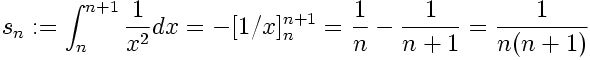if the imtermediate integral stuff looks difficult to you, just pay attention to the last part, Sn is defined as 1 over the quantity n times n plus 1.

now we can see that the first few terms of the sequence are: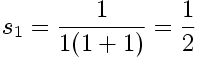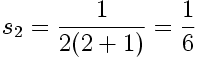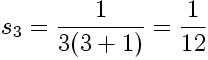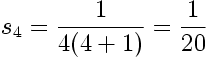does that look familiar?
it should, it is the sequence obtained from gabriel's horn in 'all about math: part I'

again, i will only include one exercise in three parts. this time, dear readers, if you have questions, please ask them in the comments instead of a personal message. remember, there is no such thing as a dumb question.

---------------------------------------------------------------------------

exercise:

(1a)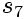(1b)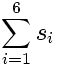(1c)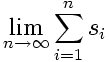i understand that in the last part of the exercise, i include a question involving limits. you can look up limits on wikipedia or ask about them in the comments.

until next time
.

POST SCRIPT NOTE: this is titled 'part II' if you must view part I, and you must, i insist.

here is a link to my myspace, which includes' Part I' of this as well as some other blogs that pertain to the show lost and any number of alternate realities pertaining to the show.

testing